Home > A2C > Chapter 9 > Lesson 9.1.3 > Problem9-59

9-59.
1. The x-intercepts of a quadratic polynomial are given below. Find a possible quadratic equation in standard form. Homework Help ✎

1.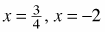2.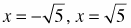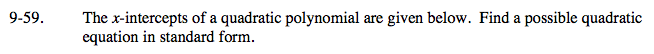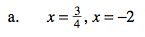y = (4x − 3)(x + 2)
y = 4x2 + 5x − 6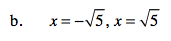y = x2 − 5